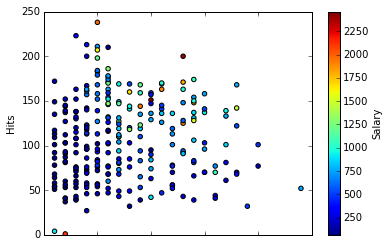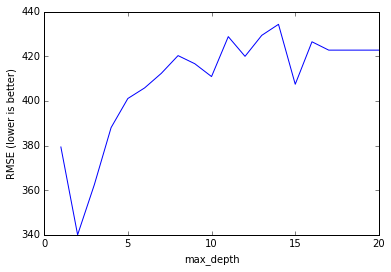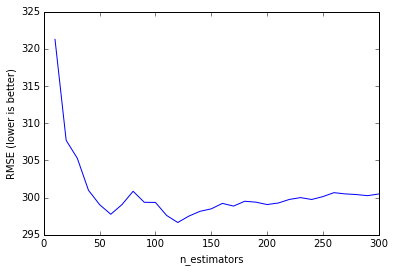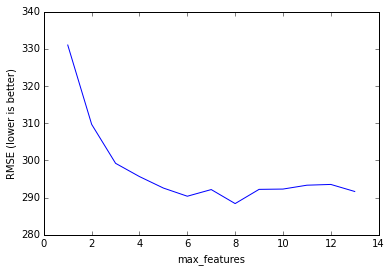# Ensembling¶

Adapted from Chapter 8 of An Introduction to Statistical Learning

Why are we learning about ensembling?

• Very popular method for improving the predictive performance of machine learning models
• Provides a foundation for understanding more sophisticated models

## Lesson objectives¶

Students will be able to:

• Define ensembling and its requirements
• Identify the two basic methods of ensembling
• Decide whether manual ensembling is a useful approach for a given problem
• Explain bagging and how it can be applied to decision trees
• Explain how out-of-bag error and feature importances are calculated from bagged trees
• Explain the difference between bagged trees and Random Forests
• Build and tune a Random Forest model in scikit-learn
• Decide whether a decision tree or a Random Forest is a better model for a given problem

# Part 1: Introduction¶

Let's pretend that instead of building a single model to solve a binary classification problem, you created five independent models, and each model was correct about 70% of the time. If you combined these models into an "ensemble" and used their majority vote as a prediction, how often would the ensemble be correct?

In :
import numpy as np

# set a seed for reproducibility
np.random.seed(1234)

# generate 1000 random numbers (between 0 and 1) for each model, representing 1000 observations
mod1 = np.random.rand(1000)
mod2 = np.random.rand(1000)
mod3 = np.random.rand(1000)
mod4 = np.random.rand(1000)
mod5 = np.random.rand(1000)

# each model independently predicts 1 (the "correct response") if random number was at least 0.3
preds1 = np.where(mod1 > 0.3, 1, 0)
preds2 = np.where(mod2 > 0.3, 1, 0)
preds3 = np.where(mod3 > 0.3, 1, 0)
preds4 = np.where(mod4 > 0.3, 1, 0)
preds5 = np.where(mod5 > 0.3, 1, 0)

# print the first 20 predictions from each model
print preds1[:20]
print preds2[:20]
print preds3[:20]
print preds4[:20]
print preds5[:20]

[0 1 1 1 1 0 0 1 1 1 1 1 1 1 1 1 1 0 1 1]
[1 1 1 1 1 1 1 0 1 0 0 0 1 1 1 0 1 0 0 0]
[1 1 1 1 0 1 1 0 0 1 1 1 1 1 1 1 1 0 1 1]
[1 1 0 0 0 0 1 1 0 1 1 1 1 1 1 0 1 1 1 0]
[0 0 1 0 0 0 1 0 1 0 0 0 1 1 1 1 1 1 1 1]

In :
# average the predictions and then round to 0 or 1
ensemble_preds = np.round((preds1 + preds2 + preds3 + preds4 + preds5)/5.0).astype(int)

# print the ensemble's first 20 predictions
print ensemble_preds[:20]

[1 1 1 1 0 0 1 0 1 1 1 1 1 1 1 1 1 0 1 1]

In :
# how accurate was each individual model?
print preds1.mean()
print preds2.mean()
print preds3.mean()
print preds4.mean()
print preds5.mean()

0.713
0.665
0.717
0.712
0.687

In :
# how accurate was the ensemble?
print ensemble_preds.mean()

0.841


Note: As you add more models to the voting process, the probability of error decreases, which is known as Condorcet's Jury Theorem.

## What is ensembling?¶

Ensemble learning (or "ensembling") is the process of combining several predictive models in order to produce a combined model that is more accurate than any individual model.

• Regression: take the average of the predictions
• Classification: take a vote and use the most common prediction, or take the average of the predicted probabilities

For ensembling to work well, the models must have the following characteristics:

• Accurate: they outperform the null model
• Independent: their predictions are generated using different processes

The big idea: If you have a collection of individually imperfect (and independent) models, the "one-off" mistakes made by each model are probably not going to be made by the rest of the models, and thus the mistakes will be discarded when averaging the models.

There are two basic methods for ensembling:

• Manually ensemble your individual models
• Use a model that ensembles for you

# Part 2: Manual ensembling¶

What makes a good manual ensemble?

• Different types of models
• Different combinations of features
• Different tuning parametersMachine learning flowchart created by the winner of Kaggle's CrowdFlower competition

## Comparing manual ensembling with a single model approach¶

• Increases predictive accuracy
• Easy to get started

• Decreases interpretability
• Takes longer to train
• Takes longer to predict
• More complex to automate and maintain
• Small gains in accuracy may not be worth the added complexity

# Part 3: Bagging¶

The primary weakness of decision trees is that they don't tend to have the best predictive accuracy. This is partially due to high variance, meaning that different splits in the training data can lead to very different trees.

Bagging is a general purpose procedure for reducing the variance of a machine learning method, but is particularly useful for decision trees. Bagging is short for bootstrap aggregation, meaning the aggregation of bootstrap samples.

What is a bootstrap sample? A random sample with replacement:

In :
# set a seed for reproducibility
np.random.seed(1)

# create an array of 1 through 20
nums = np.arange(1, 21)
print nums

# sample that array 20 times with replacement
print np.random.choice(a=nums, size=20, replace=True)

[ 1  2  3  4  5  6  7  8  9 10 11 12 13 14 15 16 17 18 19 20]
[ 6 12 13  9 10 12  6 16  1 17  2 13  8 14  7 19  6 19 12 11]


How does bagging work (for decision trees)?

1. Grow B trees using B bootstrap samples from the training data.
2. Train each tree on its bootstrap sample and make predictions.
3. Combine the predictions:
• Average the predictions for regression trees
• Take a vote for classification trees

Notes:

• Each bootstrap sample should be the same size as the original training set.
• B should be a large enough value that the error seems to have "stabilized".
• The trees are grown deep so that they have low bias/high variance.

Bagging increases predictive accuracy by reducing the variance, similar to how cross-validation reduces the variance associated with train/test split (for estimating out-of-sample error) by splitting many times an averaging the results.

## Manually implementing bagged decision trees (with B=10)¶

In :
# read in and prepare the vehicle training data
import pandas as pd
url = 'https://raw.githubusercontent.com/justmarkham/DAT8/master/data/vehicles_train.csv'
train['vtype'] = train.vtype.map({'car':0, 'truck':1})
train

Out:
price year miles doors vtype
0 22000 2012 13000 2 0
1 14000 2010 30000 2 0
2 13000 2010 73500 4 0
3 9500 2009 78000 4 0
4 9000 2007 47000 4 0
5 4000 2006 124000 2 0
6 3000 2004 177000 4 0
7 2000 2004 209000 4 1
8 3000 2003 138000 2 0
9 1900 2003 160000 4 0
10 2500 2003 190000 2 1
11 5000 2001 62000 4 0
12 1800 1999 163000 2 1
13 1300 1997 138000 4 0
In :
# set a seed for reproducibility
np.random.seed(123)

# create ten bootstrap samples (will be used to select rows from the DataFrame)
samples = [np.random.choice(a=14, size=14, replace=True) for _ in range(1, 11)]
samples

Out:
[array([13,  2, 12,  2,  6,  1,  3, 10, 11,  9,  6,  1,  0,  1]),
array([ 9,  0,  0,  9,  3, 13,  4,  0,  0,  4,  1,  7,  3,  2]),
array([ 4,  7,  2,  4,  8, 13,  0,  7,  9,  3, 12, 12,  4,  6]),
array([ 1,  5,  6, 11,  2,  1, 12,  8,  3, 10,  5,  0, 11,  2]),
array([10, 10,  6, 13,  2,  4, 11, 11, 13, 12,  4,  6, 13,  3]),
array([10,  0,  6,  4,  7, 11,  6,  7,  1, 11, 10,  5,  7,  9]),
array([ 2,  4,  8,  1, 12,  2,  1,  1,  3, 12,  5,  9,  0,  8]),
array([11,  1,  6,  3,  3, 11,  5,  9,  7,  9,  2,  3, 11,  3]),
array([ 3,  8,  6,  9,  7,  6,  3,  9,  6, 12,  6, 11,  6,  1]),
array([13, 10,  3,  4,  3,  1, 13,  0,  5,  8, 13,  6, 11,  8])]
In :
# show the rows for the first decision tree
train.iloc[samples, :]

Out:
price year miles doors vtype
13 1300 1997 138000 4 0
2 13000 2010 73500 4 0
12 1800 1999 163000 2 1
2 13000 2010 73500 4 0
6 3000 2004 177000 4 0
1 14000 2010 30000 2 0
3 9500 2009 78000 4 0
10 2500 2003 190000 2 1
11 5000 2001 62000 4 0
9 1900 2003 160000 4 0
6 3000 2004 177000 4 0
1 14000 2010 30000 2 0
0 22000 2012 13000 2 0
1 14000 2010 30000 2 0
In :
# read in and prepare the vehicle testing data
url = 'https://raw.githubusercontent.com/justmarkham/DAT8/master/data/vehicles_test.csv'
test['vtype'] = test.vtype.map({'car':0, 'truck':1})
test

Out:
price year miles doors vtype
0 3000 2003 130000 4 1
1 6000 2005 82500 4 0
2 12000 2010 60000 2 0
In :
from sklearn.tree import DecisionTreeRegressor

# grow each tree deep
treereg = DecisionTreeRegressor(max_depth=None, random_state=123)

# list for storing predicted price from each tree
predictions = []

# define testing data
X_test = test.iloc[:, 1:]
y_test = test.iloc[:, 0]

# grow one tree for each bootstrap sample and make predictions on testing data
for sample in samples:
X_train = train.iloc[sample, 1:]
y_train = train.iloc[sample, 0]
treereg.fit(X_train, y_train)
y_pred = treereg.predict(X_test)
predictions.append(y_pred)

# convert predictions from list to NumPy array
predictions = np.array(predictions)
predictions

Out:
array([[  1300.,   5000.,  14000.],
[  1300.,   1300.,  13000.],
[  2000.,   9000.,  13000.],
[  4000.,   5000.,  13000.],
[  1300.,   5000.,  13000.],
[  4000.,   5000.,  14000.],
[  4000.,   9000.,  14000.],
[  4000.,   5000.,  13000.],
[  3000.,   5000.,   9500.],
[  4000.,   5000.,   9000.]])
In :
# average predictions
np.mean(predictions, axis=0)

Out:
array([  2890.,   5430.,  12550.])
In :
# calculate RMSE
from sklearn import metrics
y_pred = np.mean(predictions, axis=0)
np.sqrt(metrics.mean_squared_error(y_test, y_pred))

Out:
461.6997581401431

## Bagged decision trees in scikit-learn (with B=500)¶

In :
# define the training and testing sets
X_train = train.iloc[:, 1:]
y_train = train.iloc[:, 0]
X_test = test.iloc[:, 1:]
y_test = test.iloc[:, 0]

In :
# instruct BaggingRegressor to use DecisionTreeRegressor as the "base estimator"
from sklearn.ensemble import BaggingRegressor
bagreg = BaggingRegressor(DecisionTreeRegressor(), n_estimators=500, bootstrap=True, oob_score=True, random_state=1)

In :
# fit and predict
bagreg.fit(X_train, y_train)
y_pred = bagreg.predict(X_test)
y_pred

Out:
array([  3351.2,   5384.4,  12971. ])
In :
# calculate RMSE
np.sqrt(metrics.mean_squared_error(y_test, y_pred))

Out:
694.05710619996307

## Estimating out-of-sample error¶

For bagged models, out-of-sample error can be estimated without using train/test split or cross-validation!

On average, each bagged tree uses about two-thirds of the observations. For each tree, the remaining observations are called "out-of-bag" observations.

In :
# show the first bootstrap sample
samples

Out:
array([13,  2, 12,  2,  6,  1,  3, 10, 11,  9,  6,  1,  0,  1])
In :
# show the "in-bag" observations for each sample
for sample in samples:
print set(sample)

set([0, 1, 2, 3, 6, 9, 10, 11, 12, 13])
set([0, 1, 2, 3, 4, 7, 9, 13])
set([0, 2, 3, 4, 6, 7, 8, 9, 12, 13])
set([0, 1, 2, 3, 5, 6, 8, 10, 11, 12])
set([2, 3, 4, 6, 10, 11, 12, 13])
set([0, 1, 4, 5, 6, 7, 9, 10, 11])
set([0, 1, 2, 3, 4, 5, 8, 9, 12])
set([1, 2, 3, 5, 6, 7, 9, 11])
set([1, 3, 6, 7, 8, 9, 11, 12])
set([0, 1, 3, 4, 5, 6, 8, 10, 11, 13])

In :
# show the "out-of-bag" observations for each sample
for sample in samples:
print sorted(set(range(14)) - set(sample))

[4, 5, 7, 8]
[5, 6, 8, 10, 11, 12]
[1, 5, 10, 11]
[4, 7, 9, 13]
[0, 1, 5, 7, 8, 9]
[2, 3, 8, 12, 13]
[6, 7, 10, 11, 13]
[0, 4, 8, 10, 12, 13]
[0, 2, 4, 5, 10, 13]
[2, 7, 9, 12]


How to calculate "out-of-bag error":

1. For every observation in the training data, predict its response value using only the trees in which that observation was out-of-bag. Average those predictions (for regression) or take a vote (for classification).
2. Compare all predictions to the actual response values in order to compute the out-of-bag error.

When B is sufficiently large, the out-of-bag error is an accurate estimate of out-of-sample error.

In :
# compute the out-of-bag R-squared score (not MSE, unfortunately!) for B=500
bagreg.oob_score_

Out:
0.7661434140978729

## Estimating feature importance¶

Bagging increases predictive accuracy, but decreases model interpretability because it's no longer possible to visualize the tree to understand the importance of each feature.

However, we can still obtain an overall summary of feature importance from bagged models:

• Bagged regression trees: calculate the total amount that MSE is decreased due to splits over a given feature, averaged over all trees
• Bagged classification trees: calculate the total amount that Gini index is decreased due to splits over a given feature, averaged over all trees

# Part 4: Random Forests¶

Random Forests is a slight variation of bagged trees that has even better performance:

• Exactly like bagging, we create an ensemble of decision trees using bootstrapped samples of the training set.
• However, when building each tree, each time a split is considered, a random sample of m features is chosen as split candidates from the full set of p features. The split is only allowed to use one of those m features.
• A new random sample of features is chosen for every single tree at every single split.
• For classification, m is typically chosen to be the square root of p.
• For regression, m is typically chosen to be somewhere between p/3 and p.

What's the point?

• Suppose there is one very strong feature in the data set. When using bagged trees, most of the trees will use that feature as the top split, resulting in an ensemble of similar trees that are highly correlated.
• Averaging highly correlated quantities does not significantly reduce variance (which is the entire goal of bagging).
• By randomly leaving out candidate features from each split, Random Forests "decorrelates" the trees, such that the averaging process can reduce the variance of the resulting model.

# Part 5: Building and tuning decision trees and Random Forests¶

• Major League Baseball player data from 1986-87: data, data dictionary (page 7)
• Each observation represents a player
• Goal: Predict player salary

## Preparing the data¶

In :
# read in the data
url = 'https://raw.githubusercontent.com/justmarkham/DAT8/master/data/hitters.csv'

# remove rows with missing values
hitters.dropna(inplace=True)

In :
hitters.head()

Out:
AtBat Hits HmRun Runs RBI Walks Years CAtBat CHits CHmRun CRuns CRBI CWalks League Division PutOuts Assists Errors Salary NewLeague
1 315 81 7 24 38 39 14 3449 835 69 321 414 375 N W 632 43 10 475.0 N
2 479 130 18 66 72 76 3 1624 457 63 224 266 263 A W 880 82 14 480.0 A
3 496 141 20 65 78 37 11 5628 1575 225 828 838 354 N E 200 11 3 500.0 N
4 321 87 10 39 42 30 2 396 101 12 48 46 33 N E 805 40 4 91.5 N
5 594 169 4 74 51 35 11 4408 1133 19 501 336 194 A W 282 421 25 750.0 A
In :
# encode categorical variables as integers
hitters['League'] = pd.factorize(hitters.League)
hitters['Division'] = pd.factorize(hitters.Division)
hitters['NewLeague'] = pd.factorize(hitters.NewLeague)

Out:
AtBat Hits HmRun Runs RBI Walks Years CAtBat CHits CHmRun CRuns CRBI CWalks League Division PutOuts Assists Errors Salary NewLeague
1 315 81 7 24 38 39 14 3449 835 69 321 414 375 0 0 632 43 10 475.0 0
2 479 130 18 66 72 76 3 1624 457 63 224 266 263 1 0 880 82 14 480.0 1
3 496 141 20 65 78 37 11 5628 1575 225 828 838 354 0 1 200 11 3 500.0 0
4 321 87 10 39 42 30 2 396 101 12 48 46 33 0 1 805 40 4 91.5 0
5 594 169 4 74 51 35 11 4408 1133 19 501 336 194 1 0 282 421 25 750.0 1
In :
# allow plots to appear in the notebook
%matplotlib inline
import matplotlib.pyplot as plt

In :
# scatter plot of Years versus Hits colored by Salary
hitters.plot(kind='scatter', x='Years', y='Hits', c='Salary', colormap='jet', xlim=(0, 25), ylim=(0, 250))

Out:
<matplotlib.axes._subplots.AxesSubplot at 0x1805e828>In :
# define features: exclude career statistics (which start with "C") and the response (Salary)
feature_cols = hitters.columns[hitters.columns.str.startswith('C') == False].drop('Salary')
feature_cols

Out:
Index([u'AtBat', u'Hits', u'HmRun', u'Runs', u'RBI', u'Walks', u'Years',
u'League', u'Division', u'PutOuts', u'Assists', u'Errors',
u'NewLeague'],
dtype='object')
In :
# define X and y
X = hitters[feature_cols]
y = hitters.Salary


## Predicting salary with a decision tree¶

Find the best max_depth for a decision tree using cross-validation:

In :
# list of values to try for max_depth
max_depth_range = range(1, 21)

# list to store the average RMSE for each value of max_depth
RMSE_scores = []

# use 10-fold cross-validation with each value of max_depth
from sklearn.cross_validation import cross_val_score
for depth in max_depth_range:
treereg = DecisionTreeRegressor(max_depth=depth, random_state=1)
MSE_scores = cross_val_score(treereg, X, y, cv=10, scoring='mean_squared_error')
RMSE_scores.append(np.mean(np.sqrt(-MSE_scores)))

In :
# plot max_depth (x-axis) versus RMSE (y-axis)
plt.plot(max_depth_range, RMSE_scores)
plt.xlabel('max_depth')
plt.ylabel('RMSE (lower is better)')

Out:
<matplotlib.text.Text at 0x18376a20>In :
# show the best RMSE and the corresponding max_depth
sorted(zip(RMSE_scores, max_depth_range))

Out:
(340.03416870475201, 2)
In :
# max_depth=2 was best, so fit a tree using that parameter
treereg = DecisionTreeRegressor(max_depth=2, random_state=1)
treereg.fit(X, y)

Out:
DecisionTreeRegressor(criterion='mse', max_depth=2, max_features=None,
max_leaf_nodes=None, min_samples_leaf=1, min_samples_split=2,
min_weight_fraction_leaf=0.0, random_state=1, splitter='best')
In :
# compute feature importances
pd.DataFrame({'feature':feature_cols, 'importance':treereg.feature_importances_}).sort('importance')

Out:
feature importance
0 AtBat 0.000000
2 HmRun 0.000000
3 Runs 0.000000
4 RBI 0.000000
5 Walks 0.000000
7 League 0.000000
8 Division 0.000000
9 PutOuts 0.000000
10 Assists 0.000000
11 Errors 0.000000
12 NewLeague 0.000000
6 Years 0.488391
1 Hits 0.511609

## Predicting salary with a Random Forest¶

In :
from sklearn.ensemble import RandomForestRegressor
rfreg = RandomForestRegressor()
rfreg

Out:
RandomForestRegressor(bootstrap=True, criterion='mse', max_depth=None,
max_features='auto', max_leaf_nodes=None, min_samples_leaf=1,
min_samples_split=2, min_weight_fraction_leaf=0.0,
n_estimators=10, n_jobs=1, oob_score=False, random_state=None,
verbose=0, warm_start=False)

### Tuning n_estimators¶

One important tuning parameter is n_estimators, which is the number of trees that should be grown. It should be a large enough value that the error seems to have "stabilized".

In :
# list of values to try for n_estimators
estimator_range = range(10, 310, 10)

# list to store the average RMSE for each value of n_estimators
RMSE_scores = []

# use 5-fold cross-validation with each value of n_estimators (WARNING: SLOW!)
for estimator in estimator_range:
rfreg = RandomForestRegressor(n_estimators=estimator, random_state=1)
MSE_scores = cross_val_score(rfreg, X, y, cv=5, scoring='mean_squared_error')
RMSE_scores.append(np.mean(np.sqrt(-MSE_scores)))

In :
# plot n_estimators (x-axis) versus RMSE (y-axis)
plt.plot(estimator_range, RMSE_scores)
plt.xlabel('n_estimators')
plt.ylabel('RMSE (lower is better)')

Out:
<matplotlib.text.Text at 0x1860e710>### Tuning max_features¶

The other important tuning parameter is max_features, which is the number of features that should be considered at each split.

In :
# list of values to try for max_features
feature_range = range(1, len(feature_cols)+1)

# list to store the average RMSE for each value of max_features
RMSE_scores = []

# use 10-fold cross-validation with each value of max_features (WARNING: SLOW!)
for feature in feature_range:
rfreg = RandomForestRegressor(n_estimators=150, max_features=feature, random_state=1)
MSE_scores = cross_val_score(rfreg, X, y, cv=10, scoring='mean_squared_error')
RMSE_scores.append(np.mean(np.sqrt(-MSE_scores)))

In :
# plot max_features (x-axis) versus RMSE (y-axis)
plt.plot(feature_range, RMSE_scores)
plt.xlabel('max_features')
plt.ylabel('RMSE (lower is better)')

Out:
<matplotlib.text.Text at 0x18d5a320>In :
# show the best RMSE and the corresponding max_features
sorted(zip(RMSE_scores, feature_range))

Out:
(288.41877774269841, 8)

### Fitting a Random Forest with the best parameters¶

In :
# max_features=8 is best and n_estimators=150 is sufficiently large
rfreg = RandomForestRegressor(n_estimators=150, max_features=8, oob_score=True, random_state=1)
rfreg.fit(X, y)

Out:
RandomForestRegressor(bootstrap=True, criterion='mse', max_depth=None,
max_features=8, max_leaf_nodes=None, min_samples_leaf=1,
min_samples_split=2, min_weight_fraction_leaf=0.0,
n_estimators=150, n_jobs=1, oob_score=True, random_state=1,
verbose=0, warm_start=False)
In :
# compute feature importances
pd.DataFrame({'feature':feature_cols, 'importance':rfreg.feature_importances_}).sort('importance')

Out:
feature importance
7 League 0.003402
12 NewLeague 0.003960
8 Division 0.007253
10 Assists 0.024857
11 Errors 0.026147
2 HmRun 0.041620
9 PutOuts 0.058637
3 Runs 0.070350
0 AtBat 0.096424
4 RBI 0.133953
1 Hits 0.143183
5 Walks 0.145255
6 Years 0.244960
In :
# compute the out-of-bag R-squared score
rfreg.oob_score_

Out:
0.53646364056364049

### Reducing X to its most important features¶

In :
# check the shape of X
X.shape

Out:
(263, 13)
In :
# set a threshold for which features to include
print rfreg.transform(X, threshold=0.1).shape
print rfreg.transform(X, threshold='mean').shape
print rfreg.transform(X, threshold='median').shape

(263L, 4L)
(263L, 5L)
(263L, 7L)

In :
# create a new feature matrix that only includes important features
X_important = rfreg.transform(X, threshold='mean')

In :
# check the RMSE for a Random Forest that only includes important features
rfreg = RandomForestRegressor(n_estimators=150, max_features=3, random_state=1)
scores = cross_val_score(rfreg, X_important, y, cv=10, scoring='mean_squared_error')
np.mean(np.sqrt(-scores))

Out:
284.82790842153145

## Comparing Random Forests with decision trees¶

• Performance is competitive with the best supervised learning methods
• Provides a more reliable estimate of feature importance
• Allows you to estimate out-of-sample error without using train/test split or cross-validation